JEE  >  BITSAT Maths Test - 5

# BITSAT Maths Test - 5

Test Description

## 45 Questions MCQ Test BITSAT Mock Tests Series & Past Year Papers | BITSAT Maths Test - 5

BITSAT Maths Test - 5 for JEE 2023 is part of BITSAT Mock Tests Series & Past Year Papers preparation. The BITSAT Maths Test - 5 questions and answers have been prepared according to the JEE exam syllabus.The BITSAT Maths Test - 5 MCQs are made for JEE 2023 Exam. Find important definitions, questions, notes, meanings, examples, exercises, MCQs and online tests for BITSAT Maths Test - 5 below.
Solutions of BITSAT Maths Test - 5 questions in English are available as part of our BITSAT Mock Tests Series & Past Year Papers for JEE & BITSAT Maths Test - 5 solutions in Hindi for BITSAT Mock Tests Series & Past Year Papers course. Download more important topics, notes, lectures and mock test series for JEE Exam by signing up for free. Attempt BITSAT Maths Test - 5 | 45 questions in 60 minutes | Mock test for JEE preparation | Free important questions MCQ to study BITSAT Mock Tests Series & Past Year Papers for JEE Exam | Download free PDF with solutions
 1 Crore+ students have signed up on EduRev. Have you?
BITSAT Maths Test - 5 - Question 1

### The area bounded by the curve y = x3, the x-axis and the ordiantes at x = -2 and x = 1 is

BITSAT Maths Test - 5 - Question 2

### If the sum of the coefficients in the expansion of (α2x2 - 2αx + 1)51 vanishes, then α is equal to

BITSAT Maths Test - 5 - Question 3

### The angle between the tangents from the origin to the circle (x-7)2 + (y+1)2 = 25 is

BITSAT Maths Test - 5 - Question 4

The limiting point of the system of co-axial circles x2+y2-6x-6y+4=0, x2+y2-2x-4y+3=0 is

BITSAT Maths Test - 5 - Question 5

If [(1 + i√3)/(1 + i√3)]n is an integer ,then n is equal to

Detailed Solution for BITSAT Maths Test - 5 - Question 5

Presuming the question actually means

[ ( 1 + √3i ) / ( 1 - √3i ) ]ⁿ

i.e. the whole thing raised to the power of n, not just the denominator.

Let w = ( 1 + √3i ) / ( 1 - √3i )

Multiplying numerator and denominator by ( 1 + √3i ) gives

w = ( 1 + √3i )² / [ ( 1 - √3i ) ( 1 + √3i ) ]

= ( 1 - 3 + 2√3i ) / ( 1 + 3 )

= ( -2 + 2√3i ) / 4

= ( -1 + √3i) / 2.

So w is a primitive cubed root of unity.     (*) ... see note below

So wⁿ is an integer

<=> wⁿ = 1

<=> n is a multiple of 3.

BITSAT Maths Test - 5 - Question 6

The differential equation for the family of curves x2 + y2 - 2ay = 0, where a is an arbitrary constant is

BITSAT Maths Test - 5 - Question 7

The number of points at which the function f(x)=|x-0.5|+|x-1|+tanx is not differentiable in (0,2) is

BITSAT Maths Test - 5 - Question 8

The solution of y'- y = 1, y(0) = 1 is given by y (x)

Detailed Solution for BITSAT Maths Test - 5 - Question 8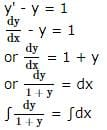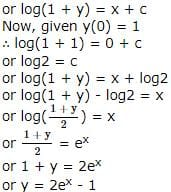BITSAT Maths Test - 5 - Question 9

The general solution of the equation x2(dy/dx)=2 is

BITSAT Maths Test - 5 - Question 10

If y = sec tan⁻1 x, then (dy/dx) =

BITSAT Maths Test - 5 - Question 11

Sum of the infinite series 1 + 3/2! + 6/3! + 10/4! + ...... is

BITSAT Maths Test - 5 - Question 12

If a flag staff of 6 mt high placed on the top of a tower throws a shadow of 2 √3 mt along the ground then the angle that the sun makes with the ground is

BITSAT Maths Test - 5 - Question 13

A variable straight line of slope 4 intersects the hyperbola xy = 1 at two points. The locus of the point which divides the line segment between these two points in the ratio 1:2 is

BITSAT Maths Test - 5 - Question 14

The general solution of the differential equation (dy/dx) = (x2/y2) is

BITSAT Maths Test - 5 - Question 15

The area bounded by the curve y2 = 9x and the lines x = 1, x = 4 and y = 0 in the first quadrant is

BITSAT Maths Test - 5 - Question 16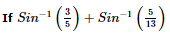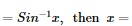BITSAT Maths Test - 5 - Question 17

Let f(x + y) = f(x)f(y) for all x and y. Suppose that f(3) = 3 and f′(0) = 11 then f′(3) is given by

BITSAT Maths Test - 5 - Question 18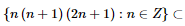BITSAT Maths Test - 5 - Question 19

A square matrix can always be expressed as a

BITSAT Maths Test - 5 - Question 20

If A and B are square matrices and A⁻1 and B⁻1 of same order exists, then (AB)⁻1 is equal to

BITSAT Maths Test - 5 - Question 21

The curved surface of the cone inscribed in a given sphere is maximum if h=

BITSAT Maths Test - 5 - Question 22

If z is a complex number, then | 3z − 1 | = 3 | z − 2 | represents

BITSAT Maths Test - 5 - Question 23

The angle between lines given by x2+4y2-7xy=0 is

BITSAT Maths Test - 5 - Question 24

If x+y+1=0 tocuhes the parabola y2=λx,then λ is equal to

BITSAT Maths Test - 5 - Question 25

The line y=mx+c touches the parabola x2=4ay if

BITSAT Maths Test - 5 - Question 26

How many words are formed from the letters of the word EAMCET so that two vowels are never together?

Detailed Solution for BITSAT Maths Test - 5 - Question 26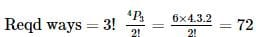BITSAT Maths Test - 5 - Question 27

Number of triangles formed by joining 12 points, no three of which are in the same straight line except 7 of them which are in a straight line, is

BITSAT Maths Test - 5 - Question 28

The mean and variance of a Binomial distribution are 6 and 4. The parameter n is

BITSAT Maths Test - 5 - Question 29

Five horses are in a race. Mr. A selects two of the horses at random and bets on them. The probability that Mr. A selected the winning horse is

Detailed Solution for BITSAT Maths Test - 5 - Question 29

Out of 5 horses only 1 is the winning horse
The probability that Mr A selected the losing horse = 4/5 x 3/4
∴ The probability that Mr A selected the winning horse = 1 - 4/5 x 3/4 = 2/5

BITSAT Maths Test - 5 - Question 30

In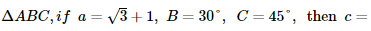BITSAT Maths Test - 5 - Question 31

In Δ A B C , i f a = 2, b = 3, c = 4, then cos A =

BITSAT Maths Test - 5 - Question 32

If the sum of the roots of the quadratic equation ax2+bx+c = 0, is equal to the sum of the squares of their reciprocals, then (a/c), (b/a), (c/b) are in

BITSAT Maths Test - 5 - Question 33

The value of 0.234 is

BITSAT Maths Test - 5 - Question 34

If a, b, c, d, e, f are in A.P. then e - c is equal to

BITSAT Maths Test - 5 - Question 35

If f (x) = (1)/(1 - x), then f[f{f (x)}] =

BITSAT Maths Test - 5 - Question 36

2 [tan⁻1(1/2) + tan⁻1(1/3) + tan⁻11] is equal to

BITSAT Maths Test - 5 - Question 37

The coordinates of mid-point of portion of line cut by coordinate axis are (3,2), the equation of the line is

BITSAT Maths Test - 5 - Question 38

A tetrahedron has vertices at O (0, 0, 0), A(1, 2, 1), B(2, 1, 3) and C (-1, 1, 2). Then the angle between the faces OAB and ABC will be

BITSAT Maths Test - 5 - Question 39

The angle between the curves y2 = x, x2 = y at (1,1) is

BITSAT Maths Test - 5 - Question 40

The direction ratios of the diagonals of a cube which joins the origin to the opposite corner are (when the 3 concurrent edges of the cube are co-ordinate axes)

BITSAT Maths Test - 5 - Question 41

In any right angled triangle hypotenuse is equal to 2√2 times the perpendicular drawn from the opposite vertex on it, then other angles are

BITSAT Maths Test - 5 - Question 42

If 2sec2α = tanβ + cotβ, then one of the value of (α+β) is

BITSAT Maths Test - 5 - Question 43

If sin 2x=n sin 2y, then the value of tan (x+y)/tan(x-y) is

Detailed Solution for BITSAT Maths Test - 5 - Question 43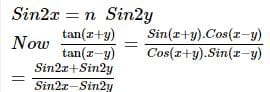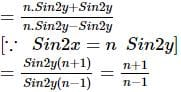BITSAT Maths Test - 5 - Question 44

The moment of force F=i+2j+3k about the point 2i-j+k is

BITSAT Maths Test - 5 - Question 45

The moment of the couple formed by the forces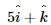a n d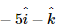acting at the points (9,−1,2) and (3,−2,1) respectively is

Detailed Solution for BITSAT Maths Test - 5 - Question 45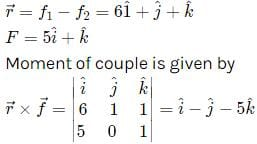## BITSAT Mock Tests Series & Past Year Papers

2 videos|15 docs|70 tests
 Use Code STAYHOME200 and get INR 200 additional OFF Use Coupon Code
Information about BITSAT Maths Test - 5 Page
In this test you can find the Exam questions for BITSAT Maths Test - 5 solved & explained in the simplest way possible. Besides giving Questions and answers for BITSAT Maths Test - 5, EduRev gives you an ample number of Online tests for practice

## BITSAT Mock Tests Series & Past Year Papers

2 videos|15 docs|70 tests